Courses

# Chapter 5 - DATA Structures, Chapter Notes, Class 12, Computer Science Class 12 Notes | EduRev

## COMPUTER SCIENCE for Class 12(XII) - CBSE & NCERT Curriculum

Created by: Imk Pathsala

## Class 12 : Chapter 5 - DATA Structures, Chapter Notes, Class 12, Computer Science Class 12 Notes | EduRev

The document Chapter 5 - DATA Structures, Chapter Notes, Class 12, Computer Science Class 12 Notes | EduRev is a part of the Class 12 Course COMPUTER SCIENCE for Class 12(XII) - CBSE & NCERT Curriculum.
All you need of Class 12 at this link: Class 12

Chapter 5 - DATA Structures, Chapter Notes, Class 12,  Computer Science

UNIT-2 DATA STRUCTURES

In Computer Science, a data structure is a particular way of storing and organizing data in a computer so
that it can be used efficiently. Different kinds of data structures are suited to different kinds of
applications, and some are highly specialized to specific tasks. For example, Stacks are used in function
call during execution of a program, while B-trees are particularly well-suited for implementation of
databases. The data structure can be classified into following two types:
Simple Data Structure: These data structures are normally built from primitive data types like integers,
floats, characters. For example arrays and structure.
Compound Data Structure: simple data structures can be combined in various ways to form more
complex structure called compound structures. Linked Lists, Stack, Queues and Trees are examples of
compound data structure.

Data Structure Arrays
Data structure array is defined as linear sequence of finite number of objects of same type with following
set of operation:

• Creating : defining an array of required size
•  Insertion: addition of a new data element in the in the array
•  Deletion: removal of a data element from the array
•  Searching: searching for the specified data from the array
•  Traversing: processing all the data elements of the array
•  Sorting : arranging data elements of the array in increasing or decreasing order
•  Merging : combining elements of two similar types of arrays to form a new array of same type

In C++ an array can be defined as
Datatype arrayname[size];
Where size defines the maximum number of elements can be hold in the array. For example
float b;//b is an array which can store maximum 10 float values
int c;
Array initialization
void main()
{i
nt b={3,5,7,8,9};//
cout<<b<<endl;
cout<<b<<endl;
}
Output is
9
0
In the above example the statement int b={3,5,7,8,9} assigns first 5 elements with the given values
and the rest elements are initialized with 0. Since in C++ index of an array starts from 0 to size-1 so the
expression b denotes the 5th element of the array which is 9 and b denotes 6th element which is
initialized with 0.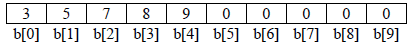We can use two different search algorithms for searching a specific data from an array

•  Linear search algorithm
•  Binary search algorithm

Linear search algorithm
In Linear search, each element of the array is compared with the given item to be searched for. This
method continues until the searched item is found or the last item is compared.
#include<iostream.h>
int linear_search(int a[], int size, int item)
{
int i=0;
while(i<size&& a[i]!=item)
i++;
if(i<size)
return i;//returns the index number of the item in the array
else
return -1;//given item is not present in the array so it returns -1 since -1 is not a legal index number
}
void main()
{
int b={2,4,5,7,8,9,12,15},size=8;
int item;
cout<<”enter a number to be searched for”;
cin>>item;
int p=linear_search(b, size, item); //search item in the array b
if(p==-1)
cout<<item<<” is not present in the array”<<endl;
else
cout<<item <<” is present in the array at index no “<<p;
}
In linear search algorithm, if the searched item is the first elements of the array then the loop terminates
after the first comparison (best case), if the searched item is the last element of the array then the loop
terminates after size time comparison (worst case) and if the searched item is middle element of the array
then the loop terminates after size/2 time comparisons (average case). For large size array linear search not an efficient algorithm but it can be used for unsorted array also.

Binary search algorithm
Binary search algorithm is applicable for already sorted array only. In this algorithm, to search for the
given item from the sorted array (in ascending order), the item is compared with the middle element of the
array. If the middle element is equal to the item then index of the middle element is returned, otherwise, if
item is less than the middle item then the item is present in first half segment of the array (i.e. between 0
to middle-1), so the next iteration will continue for first half only, if the item is larger than the middle
element then the item is present in second half of the array (i.e. between middle+1 to size-1), so the next
iteration will continue for second half segment of the array only. The same process continues until either
the item is found (search successful) or the segment is reduced to the single element and still the item is

#include<iostream.h>
int binary_search(int a[ ], int size, int item)
{
int first=0,last=size-1,middle;
while(first<=last)
{
middle=(first+last)/2;

if(item==a[middle])
return middle; // item is found
else if(item< a[middle])
last=middle-1; //item is present in left side of the middle element
else
first=middle+1; // item is present in right side of the middle element
}
return -1; //given item is not present in the array, here, -1 indicates unsuccessful search
}
void main()
{
int b={2,4,5,7,8,9,12,15},size=8;
int item;
cout<<”enter a number to be searched for”;
cin>>item;
int p=binary_search(b, size, item); //search item in the array b
if(p==-1)
cout<<item<<” is not present in the array”<<endl;
else
cout<<item <<” is present in the array at index no “<<p;
}
Let us see how this algorithm work for item=12
Initializing first =0 ; last=size-1; where size=8
Iteration 1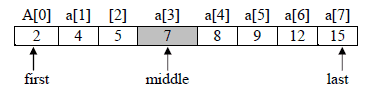First=0, last=7
middle=(first+last)/2=(0+7)/2=3 // note integer division 3.5 becomes 3
value of a[middle] i.e. a is 7
7<12 then first= middle+1 i.e. 3 + 1 =4
iteration 2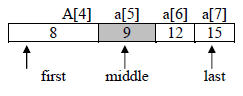first=4, last=7
middle=(first+last)/2=(4+7)/2=5
value of a[middle] i.e. a is 9
9<12 then first=middle+1;5+1=6
iteration 3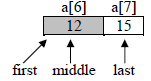first=6,last=7

middle=(first+last)/2 = (6+7)/2=6
value of a[middle] i.e. a is 12 which is equal to the value of item being search i.e.12
As a successful search the function binary_search() will return to the main function with value 6 as
index of 12 in the given array. In main function p hold the return index number.

Note that each iteration of the algorithm divides the given array in to two equal segments and the only one
segment is compared for the search of the given item in the next iteration. For a given array of size N= 2n
elements, maximum n number of iterations are required to make sure whether the given item is present in
the given array or not, where as the linear requires maximum 2n number of iteration. For example, the
number of iteration required to search an item in the given array of 1000 elements, binary search requires
maximum 10 (as 1000210) iterations where as linear search requires maximum 1000 iterations.

Inserting a new element in an array
We can insert a new element in an array in two ways

•  If the array is unordered, the new element is inserted at the end of the array
•  If the array is sorted then the new element is added at appropriate position without altering the order. To achieve this, all elements greater than the new element are shifted. For example, to add 10 in the given array below: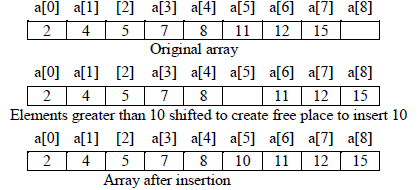Following program implement insertion operation for sorted array

#include<iostream.h>
void insert(int a[ ], int &n, int item) //n is the number of elements already present in the array
{
int i=n-1;
while (i>=0 && a[i]>item)
{
a[i+1]=a[i]; // shift the ith element one position towards right
i--;
}
a[i+1]=item; //insertion of item at appropriate place
n++; //after insertion, number of elements present in the array is increased by 1
}
void main()
{int a={2,4,5,7,8,11,12,15},n=8;
int i=0;
cout<<“Original array is: ”;
for(i=0;i<n;i++)
cout<<a[i]<<”, “;
insert(a,n,10);
cout<<” Array after inserting 10 is: ”;

for(i=0; i<n; i++)
cout<<a[i]<<”, “;
}

Output is

Original array is:
2, 4, 5, 7, 8, 11, 12, 15
Array after inserting 10 is:
2, 4, 5, 7, 8, 10, 11, 12, 15

Deletion of an item from a sorted array
In this algorithm the item to be deleted from the sorted array is searched and if the item is found in the
array then the element is removed and the rest of the elements are shifted one position toward left in the
array to keep the ordered array undisturbed. Deletion operation reduces the number of elements present in
the array by1. For example, to remove 11 from the given array below: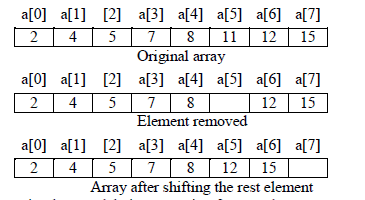Following program implement deletion operation for sorted array
#include<iostream.h>
void delete_item(int a[ ], int &n, int item) //n is the number of elements already present in the array
{int i=0;
while(i<n && a[i]<item)
i++;
if (a[i]==item) // given item is found
{while (i<n)
{a[i]=a[i+1]; // shift the (i+1)th element one position towards left
i++;
}
cout<<” Given item is successfully deleted”;
}
else
n--;
}
void main()
{int a={2,4,5,7,8,11,12,15},n=8;
int i=0;
cout<<“Original array is : ”;
for(i=0;i<n;i++)
cout<<a[i]<<”, “;
delete_item(a,n,11);
cout<<” Array after deleting 11 is: ”;
for(i=0; i<n; i++)
cout<<a[i]<<”, “;
}
Output is
Original array is:
2, 4, 5, 7, 8, 11, 12, 15
Given item is successfully deleted
Array after deleting 11 is:
2, 4, 5, 7, 8, 12, 15

Traversal
Processing of all elements (i.e. from first element to the last element) present in one-dimensional array is
called traversal. For example, printing all elements of an array, finding sum of all elements present in an
array.

#include<iostream.h>
void print_array(int a[ ], int n) //n is the number of elements present in the array
{int i;
cout<<” Given array is : ”;
for(i=0; i<n; i++)
cout<<a[i]<<”, “;
}
int sum(int a[ ], int n)
{int i,s=0;
for(i=0; i<n; i++)
s=s+a[i];
return s;
}
void main()
{int b={3,5,6,2,8,4,1,12,25,13},n=10;
int i, s;
print_array(b,n);
s=sum(b,n);
cout<<” Sum of all elements of the given array is : ”<<s;
}

Output is
Given array is
3, 5, 6, 2, 8, 4, 1, 12, 25, 13
Sum of all elements of the given array is : 79

Sorting
The process of arranging the array elements in increasing (ascending) or decreasing (descending) order is known as sorting. There are several sorting techniques are available e.g. selection sort, insertion sort,
bubble sort, quick sort, heap short etc. But in CBSE syllabus only selection sort, insertion sort, bubble sort
are specified.

Selection Sort
The basic idea of a selection sort is to repeatedly select the smallest element in the remaining unsorted
array and exchange the selected smallest element with the first element of the unsorted array. For
example, consider the following unsorted array to be sorted using selection sort

Original array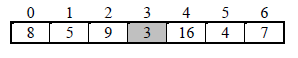iteration 1 : Select the smallest element from unsorted array which is 3 and exchange 3 with the first
element of the unsorted array i.e. exchange 3 with 8. After iteration 1 the element 3 is at its
final position in the array.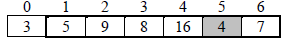Iteration 2: The second pass identify 4 as the smallest element and then exchange 4 with 5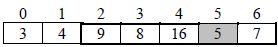Iteration 3: The third pass identify 5 as the smallest element and then exchange 5 with 9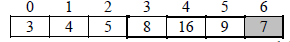Iteration 4: The third pass identify 7 as the smallest element and then exchange 7 with 8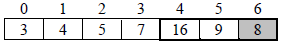Iteration 5: The third pass identify 8 as the smallest element and then exchange 8 with 16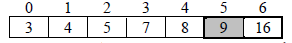Iteration 6: The third pass identify 9 as the smallest element and then exchange 9 with 9 which makes
no effect.

The unsorted array with only one element i.e. 16 is already at its appropriate position so no more iteration
is required. Hence to sort n numbers, the number of iterations required is n-1, where in each next iteration,
the number of comparison required to find the smallest element is decreases by 1 as in each pass one
element is selected from the unsorted part of the array and moved at the end of sorted part of the array .
For n=7 the total number of comparison required is calculated as
Pass1: 6 comparisons i.e. (n-1)
Pass2: 5 comparisons i.e. (n-2)
Pass3: 4 comparisons i.e. (n-3)
Pass4: 3 comparisons i.e. (n-4)
Pass5: 2 comparisons i.e. (n-5)
Pass6: 1 comparison i.e. (n-6)=(n-(n-1))
Total comparison for n=(n-1)+(n-2)+(n-3)+ ……….+(n-(n-1))= n(n-1)/2
7=6+5+4+3+2+1=7*6/2=21;
Note: For given array of n elements, selection sort always executes n(n-1)/2 comparison statements
irrespective of whether the input array is already sorted(best case), partially sorted(average case) or
totally unsorted(i.e. in reverse order)(worst case).
#include<iostream.h>
void select_sort(int a[ ], int n) //n is the number of elements present in the array
{int i, j, p, small;
for(i=0;i<n-1;i++)
{small=a[i]; // initialize small with the first element of unsorted part of the array
p=i; // keep index of the smallest number of unsorted part of the array in p
66
for(j=i+1; j<n; j++) //loop for selecting the smallest element form unsorted array
{if(a[j]<small)
{small=a[j];
p=j;
}
}// end of inner loop----------
//----------exchange the smallest element with ith element-------------
a[p]=a[i];
a[i]=small;
//-----------end of exchange-------------
}
}//end of function
void main( )
{int a={8,5,9,3,16,4,7},n=7,i;
cout<<” Original array is : ”;
for(i=0;i<n;i++)
cout<<a[i]<<”, “;
select_sort(a,n);
cout<<” The sorted array is: ”;
for(i=0; i<n; i++)
cout<<a[i]<<”, “;
}
Output is
Original array is
8, 5, 9, 3, 16, 4, 7
The sorted array is
3, 4, 5, 7, 8, 9, 16

Insertion Sort
Insertion sort algorithm divides the array of n elements in to two subparts, the first subpart contain a to
a[k] elements in sorted order and the second subpart contain a[k+1] to a[n] which are to be sorted. The
algorithm starts with only first element in the sorted subpart because array of one element is itself in
sorted order. In each pass, the first element of the unsorted subpart is removed and is inserted at the
appropriate position in the sorted array so that the sorted array remain in sorted order and hence in each
pass the size of the sorted subpart is increased by 1 and size of unsorted subpart is decreased by 1. This
process continues until all n-1 elements of the unsorted arrays are inserted at their appropriate position in
the sorted array.

For example, consider the following unsorted array to be sorted using selection sort

Original array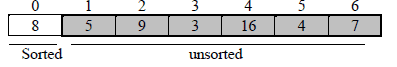Sorted unsorted
Initially the sorted subpart contains only one element i.e. 8 and the unsorted subpart contains n-1 elements
where n is the number of elements in the given array.

Iteration1: To insert first element of the unsorted subpart i.e. 5 into the sorted subpart, 5 is compared
with all elements of the sorted subpart starting from rightmost element to the leftmost
element whose value is greater than 5, shift all elements of the sorted subpart whose value
is greater than 5 one position towards right to create an empty place at the appropriate position in the sorted array, store 5 at the created empty place, here 8 will move from position a to a and a is filled by 5. After first pass the status of the array is: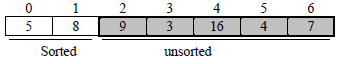Iteration2: In second pass 9 is the first element of the unsorted subpart, 9 is compared with 8, since 8
is less than 9 so no shifting takes place and the comparing loop terminates. So the element
9 is added at the rightmost end of the sorted subpart. After second pass the status of the
array is: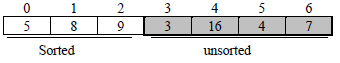Iteration3: in third pass 3 is compared with 9, 8 and 5 and shift them one position towards right and
insert 3 at position a. After third pass the status of the array is: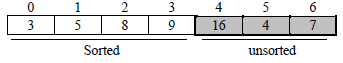Iteration4: in forth pass 16 is greater than the largest number of the sorted subpart so it remains at the
same position in the array. After fourth pass the status of the array is: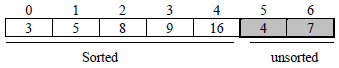Iteration5: in fifth pass 4 is inserted after 3. After third pass the status of the array is: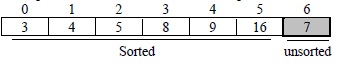Iteration6: in sixth pass 7 is inserted after 5. After fifth pass the status of the array is: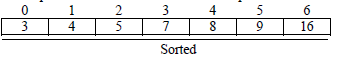Insertion sort take advantage of sorted(best case) or partially sorted(average case) array because if all
elements are at their right place then in each pass only one comparison is required to make sure that the
element is at its right position. So for n=7 only 6 (i.e. n-1) iterations are required and in each iteration only
one comparison is required i.e. total number of comparisons required= (n-1)=6 which is better than the
selection sort (for sorted array selection sort required n(n-1)/2 comparisons). Therefore insertion sort is
best suited for sorted or partially sorted arrays.
#include<iostream.h>
void insert_sort(int a[ ],int n) //n is the no of elements present in the array
{int i, j,p;
for (i=1; i<n; i++)
{p=a[i];
j=i-1;
//inner loop to shift all elements of sorted subpart one position towards right
0 1 2 3 4 5 6
3 5 8 9 16 4 7
68
while(j>=0&&a[j]>p)
{
a[j+1]=a[j];
j--;
}
//---------end of inner loop
a[j+1]=p; //insert p in the sorted subpart
}
}
void main( )
{
int a={8,5,9,3,16,4,7},n=7,i;
cout<<” Original array is : ”;
for(i=0;i<n;i++)
cout<<a[i]<<”, “;
insert_sort(a,n);
cout<<” The sorted array is: ”;
for(i=0; i<n; i++)
cout<<a[i]<<”, “;
}

Output is
Original array is
8, 5, 9, 3, 16, 4, 7
The sorted array is
3, 4, 5, 7, 8, 9, 16

Bubble Sort
Bubble sort compares a[i] with a[i+1] for all i=0..n-2, if a[i] and a[i+1] are not in ascending order then
exchange a[i] with a[i+1] immediately. After each iteration all elements which are not at their proper
position move at least one position towards their right place in the array. The process continues until all
elements get their proper place in the array (i.e. algorithm terminates if no exchange occurs in the last
iteration)
For example, consider the following unsorted array to be sorted using selection sort
Original array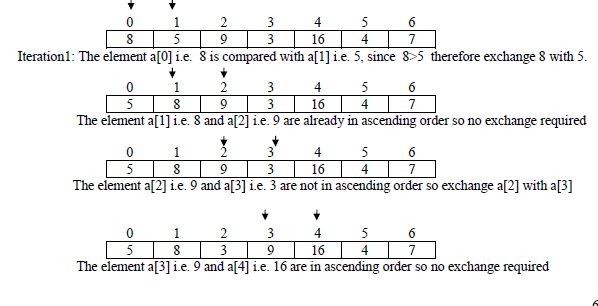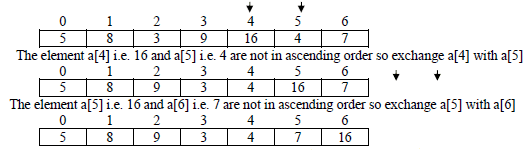Since in iteration1 some elements were exchanged with each other which shows that array
was not sorted yet, next iteration continues. The algorithm will terminate only if the last
iteration do not process any exchange operation which assure that all elements of the array
are in proper order.
Iteration2: only exchange operations are shown in each pass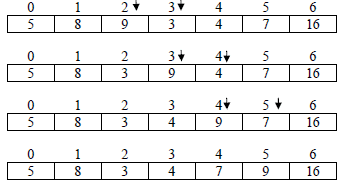In iteration 2 some exchange operations were processed, so, at least one more iteration is
required to assure that array is in sorted order.

Iteration3: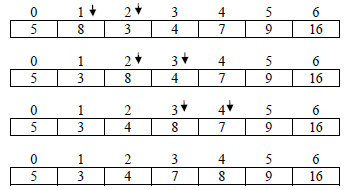Iteration4: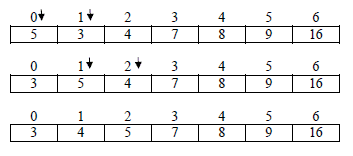Iteration5: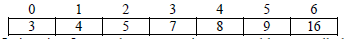In iteration 5 no exchange operation executed because all elements are already in proper
order therefore the algorithm will terminate after 5th iteration.

Merging of two sorted arrays into third array in sorted order
Algorithm to merge arrays a[m](sorted in ascending order) and b[n](sorted in descending order) into third
array C[n+m] in ascending order.

#include<iostream.h>
Merge(int a[ ], int m, int b[n], int c[ ])// m is size of array a and n is the size of array b
{int i=0; // i points to the smallest element of the array a which is at index 0
int j=n-1;// j points to the smallest element of the array b which is at the index m-1 since b is
// sortet in descending order
int k=0; //k points to the first element of the array c
while(i<m&&j>=0)
{if(a[i]<b[j])
c[k++]=a[i++]; // copy from array a into array c and then increment i and k
else
c[k++]=b[j--]; // copy from array b into array c and then decrement j and increment k
}
while(i<m) //copy all remaining elements of array a
c[k++]=a[i++];
while(j>=0) //copy all remaining elements of array b
c[k++]=b[j--];
}
void main()
{int a={2,4,5,6,7},m=5; //a is in ascending order
int b={15,12,4,3,2,1},n=6; //b is in descending order
int c;
merge(a, m, b, n, c);
cout<<”The merged array is : ”;
for(int i=0; i<m+n; i++)
cout<<c[i]<”, “;
}
Output is
The merged array is:
1, 2, 2, 3, 4, 4, 5, 6, 7, 12, 15

Two dimensional arrays
In computing, row-major order and column-major order describe methods for storing multidimensional
arrays in linear memory. Following standard matrix notation, rows are identified by the first index of a
two-dimensional array and columns by the second index. Array layout is critical for correctly passing
arrays between programs written in different languages. Row-major order is used in C, C++; columnmajor
order is used in Fortran and MATLAB.

Row-major order
In row-major storage, a multidimensional array in linear memory is accessed such that rows are stored one
after the other. When using row-major order, the difference between addresses of array cells in increasing
rows is larger than addresses of cells in increasing columns. For example, consider this 2×3 array: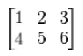An array declared in C as
int A = { {1, 2, 3}, {4, 5, 6} };
would be laid out contiguously in linear memory as:

1 2 3 4 5 6

To traverse this array in the order in which it is laid out in memory, one would use the following nested
loop:
for (i = 0; i < 2; i++)
for (j = 0; j < 3; j++)
cout<<A[i][j];
The difference in offset from one column to the next is 1*sizeof(type) and from one row to the next is
3*sizeof(type). The linear offset from the beginning of the array to any given element A[row][column]
can then be computed as:
offset = row*NUMCOLS + column
Address of element A[row][column] can be computed as:
Address of A[row][column]=base address of A + (row*NUMCOLS + column)* sizeof (type)
Where NUMCOLS is the number of columns in the array.
The above formula only works when using the C, C++ convention of labeling the first element 0. In other
words, row 1, column 2 in matrix A, would be represented as A
Note that this technique generalizes, so a 2×2×2 array looks like:
int A = {{{1,2}, {3,4}}, {{5,6}, {7,8}}};
and the array would be laid out in linear memory as:

1 2 3 4 5 6 7 8

Example 1.
For a given array A is stored in the memory along the row with each of its elements occupying 4
bytes. Calculate address of A if the base address of array A is 5000.
Solution:
For given array A[M][N] where M=Number of rows, N =Number of Columns present in the array
here M=10, N=20, I=3, J=5, sizeof(type)=4 bytes
address of A = 5000 + (3 * 20 + 5) * 4
= 5000 + 65*4=5000+260=5260

Example 2.
An array A is stored in the memory along the row with each of its elements occupying 8 bytes.
Find out the location of A, if A is stored at 4000.
Solution:
For given array A[M][N] where M=Number of rows, N =Number of Columns present in the array
here M=50, N=20, sizeof(type)=8, I=4, J=5
4000 = base address + 85*8
Now to find address of A
here M=50, N=20, sizeof(type)=8, I=5, J=10
=3320 + 110*8 = 3320+880 = 4200
As C, C++ supports n dimensional arrays along the row, the address calculation formula can be
generalized for n dimensional array as:
For 3 dimentional array A[m][n][p], find address of a[i][j][k]:
Address of a[i][j][k] = base address + ( (I * n + j ) * p + k ) * sizeof(type)
For 4 dimentional array A[m][n][p][q], find address of a[i][j][k][l]:
Address of a[i][j][k][l] = base address + ( ( (I * n + j ) * p + k ) * p + l ) * sizeof(type)
Column-major order is a similar method of flattening arrays onto linear memory, but the columns are
listed in sequence. The programming languages Fortran, MATLAB, use column-major ordering. The
arrayif stored contiguously in linear memory with column-major order would look like the following:

1 4 2 5 3 6

The memory offset could then be computed as:
offset = row + column*NUMROWS

Address of element A[row][column] can be computed as:

Where NUMROWS represents the number of rows in the array in this case, 2.
Treating a row-major array as a column-major array is the same as transposing it. Because performing a
transpose requires data movement, and is quite difficult to do in-place for non-square matrices, such
transpositions are rarely performed explicitly. For example, software libraries for linear algebra, such as
the BLAS, typically provide options to specify that certain matrices are to be interpreted in transposed
order to avoid the necessity of data movement

Example1.
For a given array A is stored in the memory along the column with each of its elements occupying
4 bytes. Calculate address of A if the base address of array A is 5000.
Solution:
For given array A[M][N] where M=Number of rows, N =Number of Columns present in the array
here M=10, N=20, I=3, J=5, sizeof(type)=4 bytes
Address of A = 5000 + (5 * 10 + 3) * 4
= 5000 + 53*4 = 5000+215 =5215

Example2.
An array A is stored in the memory along the column with each of its elements occupying 8 bytes.
Find out the location of A, if A is stored at 4000.
Solution:
For given array A[M][N] where M=Number of rows, N =Number of Columns present in the array
here M=50, N=20, sizeof(type)=8, I=4, J=5
4000 = base address + 254*8
Now to find address of A
here M=50, N=20, sizeof(type)=8, I =5, J=10
=1968 + 510*8 = 1968+4080 = 6048

9 docs

,

,

,

,

,

,

,

,

,

,

,

,

,

,

,

,

,

,

,

,

,

,

,

,

,

,

,

,

,

,

;